# Period mapping

(diff) ← Older revision | Latest revision (diff) | Newer revision → (diff)

A mapping which assigns to a pointof the baseof a familyof algebraic varieties over the fieldof complex numbers the cohomology spacesof the fibre over this point, provided with a Hodge structure. The Hodge structure thus obtained is considered as a point in the moduli variety of Hodge structures of a given type.

The study of period mappings dates back to the studies of N.H. Abel and C.G.J. Jacobi on integrals of algebraic functions (see Abelian differential). However, until recently, the only period mappings that have been studied were those which correspond to families of curves.

Let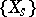be the family of fibres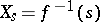of a smooth projective morphism, whereis a smooth variety. The cohomology spaces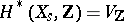are then provided with a pure polarized Hodge structure, which is defined by a homomorphism of real algebraic groups (cf. Algebraic group), where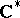is the multiplicative group of the field of complex numbers, considered as a real algebraic group, while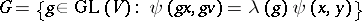is the algebraic group of linear transformations of a spacethat multiply a non-singular (symmetric or skew-symmetric) bilinear formby a scalar factor; the automorphism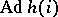of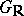is thus a Cartan involution and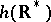lies in the centre of. The set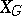of homomorphismswhich possess the above properties is naturally provided with the-invariant structure of a homogeneous Kähler manifold and is called a Griffiths variety, while the quotient varietyis the moduli space of the Hodge structures. The homomorphismdefines the Hodge decomposition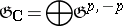of the Lie algebra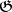of the group, where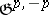is the subspace in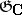on which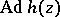operates by multiplication by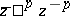. The assignment, where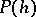is the parabolic subgroup in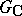with Lie algebra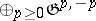, defines an open dense imbedding of the varietyinto the compact-homogeneous flag manifold. In the tangent space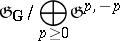toat the point, the horizontal subspaceis distinguished. A holomorphic mapping intooris said to be horizontal if the image of its tangential mapping lies in a horizontal subbundle.

It has been established that the period mapping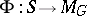is horizontal (see , ). The singularities of period mappings are described by the Schmid nilpotent orbit theorem, which, whenis a curve with a deleted point, asserts that ifis the local coordinate on,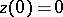, then when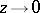,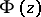is asymptotically close to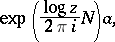whereand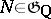is a nilpotent element (see ). The image of the monodromy group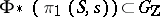is semi-simple in every rational representation of the group, while transference ofaround a divisor with normal intersections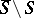in a smooth compactificationof the varietygenerates quasi-unipotent elements(i.e. elements which take roots of unity as eigen values). The importance of the monodromy group is underlined by the rigidity theorem (see , , ): If there are two families of algebraic varieties over, then the relevant period mappings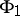and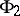frominto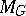coincide if and only ifat a certain point, and if the homomorphisms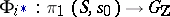,, coincide.

Complete results on the structure of the kernel and the image of a period mapping generally relate to the cases of curves and-surfaces (cf.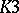-surface). Ifis a family of varieties of the type indicated and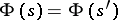, then(Torelli's theorem), while for-surfaces the maximum possible image of the period mapping coincides with(see ). In the case of curves, the image of the period mapping has been described partially (Schottky–Yung relations, see , ). The Griffiths conjecture states that a moduli variety permits a partial analytic compactification, i.e. an open imbedding in an analytic space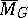such that the period mapping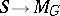can be continued to a holomorphic mapping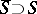for every smooth compactification. Such a compactification is known (1983) only for the case whereis a symmetric domain .

How to Cite This Entry:
Period mapping. Encyclopedia of Mathematics. URL: http://encyclopediaofmath.org/index.php?title=Period_mapping&oldid=16699
This article was adapted from an original article by A.I. Ovseevich (originator), which appeared in Encyclopedia of Mathematics - ISBN 1402006098. See original article